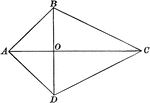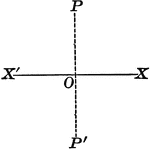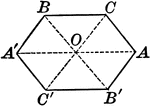Axis of Symmetry Drawn on a Hexagon

Illustration of an axis of symmetry drawn with respect to a hexagon.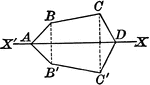Axis of Symmetry Drawn on a Hexagon

Illustration of an axis of symmetry drawn with respect to a hexagon.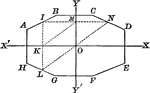Octagon with Symmetry

Illustration of an octagon with symmetry with respect to two axes perpendicular to each other. It is…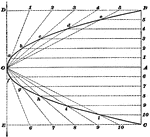Construction Of Parabola

Illustration used to show how to draw a parabola when the axis and longest double ordinate is given.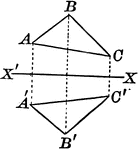Axis of Symmetry Drawn on a Quadrilateral

Illustration of an axis of symmetry drawn with respect to a quadrilateral.